D3 Range
FREE     4 minute and 0 seconds read

Takeaways:
n/a
Resources:
n/a
D3 Tutorial:

D3 Range

D3 Range Introduction

D3 Range is part of the D3.js data visualizations library.

The D3 Range function helps you go from your input data to a data representation that will fit into the space in which you want to create your chart.

We'll use D3.js version 5.9.7 for this D3 Range example.

``````d3.version;
``````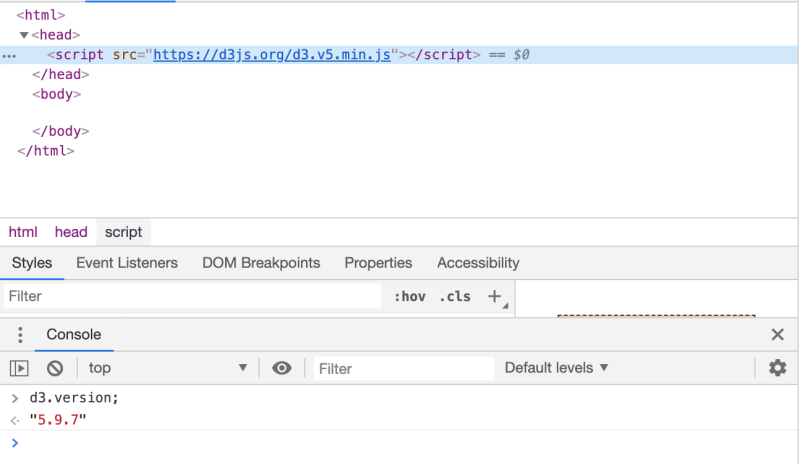D3 Range Context Explanation

D3 Range comes into play when using the D3 Scale.

D3 Range is how you tell D3 what the input domain that you fed into your D3 Scale needs to be transformed into.

Let's look at two D3 Range examples:

D3 Range Example One

D3 Range can be used to allow you to make your small input data larger.

Let's say your input data is very small as in 0.000100, 0.0001001, 0.0001002, 0.0001003 all the way through 0.0002000.

To represent this on a web page D3 chart, you need to be able to somehow translate those very small numbers into pixels that are visible on your web page.

Whether you create your dashboard with SVG or Canvas or HTML elements, you need a way to make your small numbers bigger.

You do that with the D3 Scale.

Let's define a D3 scale and tell D3 that while our input data covers the values from 0.000100 to 0.000200, we want it to be represented as going from 0 to 100.

``````var d3_range_example_scale = d3.scaleLinear()
.domain([0.000100, 0.000200])
.range( [0,        100     ]);
``````

Notice that the D3 Range function is where you pass an array with what you want the final values of your data to be converted into.

The left most value in the range array, 0, will be the transformed representation of 0.000100 and the right most value in the range array, 100, will be the transformed representation of 0.000200.

Now that we have the D3 Range set and the D3 scale defined, let's pass in values into the D3 example scale to see what we get.

First, we pass in the left most data value -> 0.000100

``````d3_range_example_scale(0.000100)
``````

And we can see the D3 Scale function returns the value 0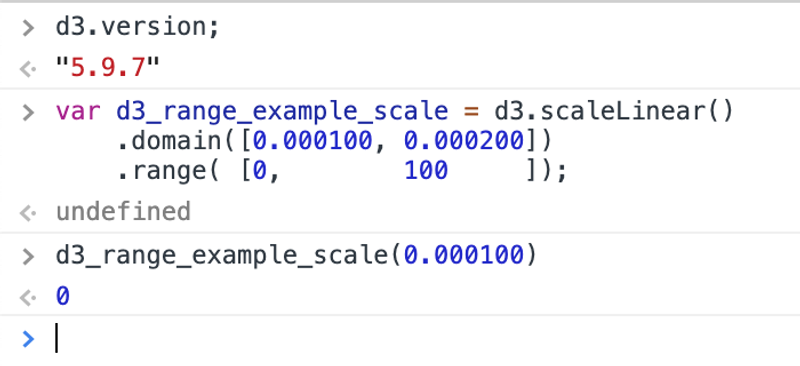Next, we pass in the right most data value -> 0.000200

``````d3_range_example_scale(0.000200)
``````

And we can see the D3 Scale function returns the value 100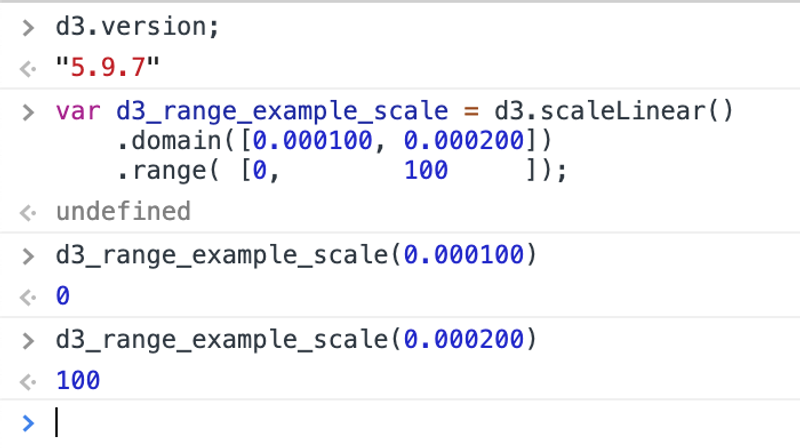Last, we pass in a value in the middle to see if the extrapolation works -> 0.000150

``````d3_range_example_scale(0.000150)
``````

And we can see the D3 Scale function returns the value 50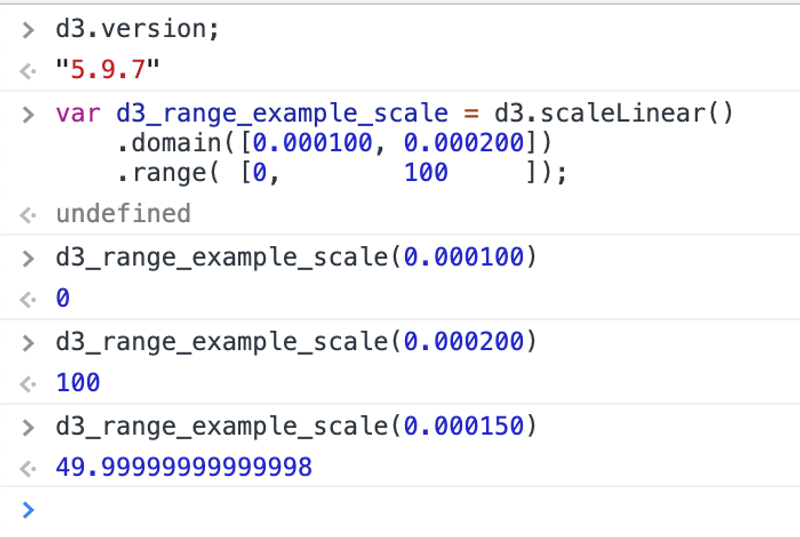Perfect - you were able to use D3 Range to go from small data to a bigger data representation for your chart.

D3 Range Example Two

D3 Range can be used to allow you to make your large input data readable.

Let's say your input data is large as in 1000, 1001, 1002, 1003 all the way through 2000.

To represent this on a web page D3 chart, you need to be able to somehow translate those large numbers into pixels that are visible on your web page.

Whether you create your dashboard with SVG or Canvas or HTML elements, you need a way to make your large numbers smaller.

You do that with the D3 Scale.

Let's define a D3 scale and tell D3 that while our input data covers the values from 1000 to 2000, we want it to be represented as going from 0 to 100.

``````var d3_range_example_scale = d3.scaleLinear()
.domain([1000, 2000])
.range( [   0,  100]);
``````

Notice that the D3 Range function is where you pass an array with what you want the final values of your data to be converted into.

The left most value in the range array, 0, will be the transformed representation of 1000 and the right most value in the range array, 100, will be the transformed representation of 2000.

Now that we have the D3 Range set and the D3 scale defined, let's pass in values into the D3 Range example scale to see what we get.

First, we pass in the left most data value -> 1000

``````d3_range_example_scale(1000)
``````

And we can see the D3 Scale function returns the value 0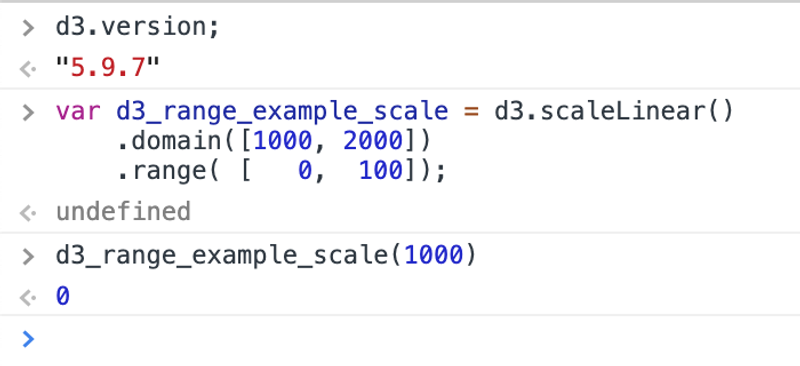Next, we pass in the right most data value -> 2000

``````d3_range_example_scale(2000)
``````

And we can see the D3 Scale function returns the value 100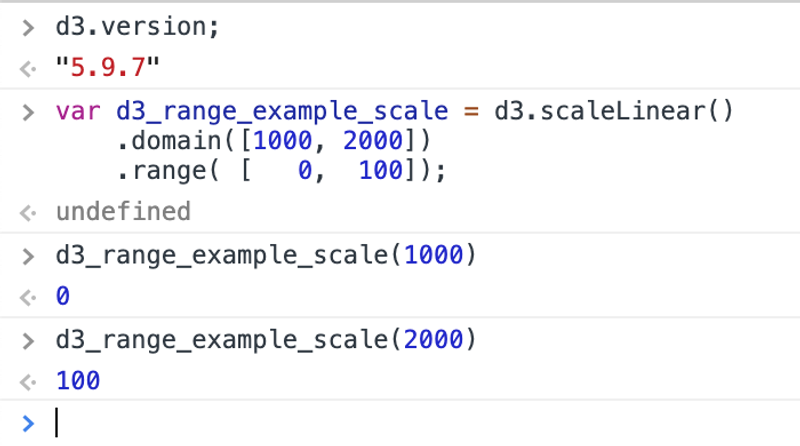Last, we pass in a value in the middle to see if the extrapolation works -> 1500

``````d3_range_example_scale(1500)
``````

And we can see the D3 Scale function returns the value 50Perfect - you were able to use D3 Range to go from large to a smaller data representation for your chart.

Next steps with D3 Range

Now that you've seen how the D3 Range function helps you go from your input data to a data representation that will fit into the space in which you want to create your chart, you'll want to try it own your own.

If you have any questions or want clarification on any of the above, please email me at sebastian@dashingd3js.com.

<< Back To D3 Screencast and Written Tutorials Index